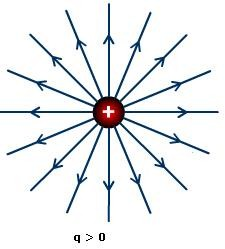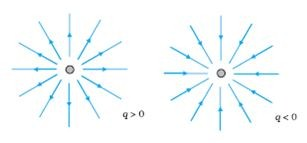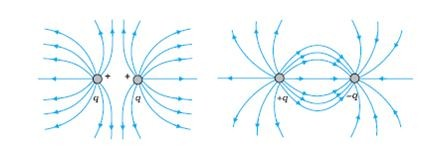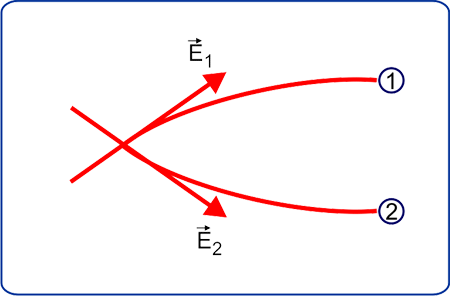Click to Chat

1800-1023-196

+91-120-4616500

CART 0

• 0

MY CART (5)

Use Coupon: CART20 and get 20% off on all online Study Material

ITEM
DETAILS
MRP
DISCOUNT
FINAL PRICE
Total Price: Rs.

There are no items in this cart.
Continue Shopping• Complete Physics Course - Class 11
• OFFERED PRICE: Rs. 2,968
• View Details

```Electric Field Lines

Table of Content

Introduction to Electric Field Lines

Electric Field Lines

Properties of Electric Field Lines

Introduction to Electric Field Lines

As electric field, that is, force per unit charge is a vector quantity; it can be used to represent overall effect of electric field in system of electric charges. Similarly electric field can be used in pictorial form to describe the overall intensity of the field. This pictorial representation or form is called electric field lines. There are certain properties, rules and applications of electric field lines. The idea behind utilizing electric field lines is to represent nature of electric field and making it simpler to apply in complex physics problems.

Electric Field Lines

Electric Field Lines can be defined as a curve which shows direction of electric field, when we draw tangent at its point. The concept of electric field was proposed by Michael Faraday, in the 19th century. He always thought that electric field lines can be used to describe and interpret the invisible electric field. Instead of using complex vector diagram every time, electric field lines can be used to describe electric field around a system of charges in a better way.

Example: Consider a charge ‘q’, placed at origin. The electric field E produced by charge q can be represented as:Image 1: Electric field lines in case of positive charge

The strength of electric fields is directly proportional to the lengths of electric field lines. Also, since electric field is inversely proportional to square of distance, the electric field strength decreases, as we move away from the charge. The direction of arrows of field lines depicts direction of electric field, which is pointing outwards in case of positive charge and pointing inwards in case of negative charge.Image 2: Electric field lines in case of negative charge

Adding further, the magnitude of electric field is well described by the density of charges. The lines closer to charge represent a strong electric field and the lines away from charge correspond to weak electric field. This is because, the strength of electric field decreases as we move away from charge. It is not necessary to draw as many electric field lines in order to key out the electric field around the charge ‘q’. Even if we draw few lines along the charge, we can trace the nature of electric field by judging the relative density of field lines.

Properties of Electric Field Lines

Electric field lines show some general properties, to account for nature of electric fields. Some general properties of electric field lines are as follows:

Electric field lines start from positive charge and close at negative charge, in case of a single charge, electric field end at infinityImage 3: Electric field lines are away from positive charge and towards negative charge

In a charge free- region, we can electric field lines to be continuous and smooth​

Image 4: Electric field lines start from positive charge and terminate on negative charge

Two electric field lines never intersect or cross each other, as if they do, there will be two vectors depicting two directions of the same electric field, which is not possible

Electric field lines never form a closed loop of lines, as electric field is conservative in nature, so they are of open natureImage 5: Electric field lines never form closed loops

The electric field lines are always normal in the direction of electric field

Magnitude of electric field is represented by density of electric field lines

Electric field lines are imaginary in nature, they are simply used to represent pictorial form of electric field lines

We if draw a tangent at any point of electric field line, we get direction of electric field

The electric field lines are closer where electric field is strong, and farther, where the strength of electric field is weak

Electric field lines are conductor-resistance that is they can’t pass through a conductor. Thereby making conductor free from influence of electric field

Electric field lines can pass through an insulator

The number of electric field lines per unit cross-sectional area is directly proportional to electric field strength of that area

Electric field lines exert lateral pressure on each other, to show force of repulsion

Electric field lines contract longitudinally

Example: Why electric field lines never intersect each other?

Electric field lines tell the nature of electric field in a system of charges. They also reveal about direction of electric field strength at a point in a region of space. If the electric field lines intersect, then there must be distinctly two electric fields with different directions. This is not possible as a single location has only one direction and magnitude of electric field associated with it. The figure below describes it well.Image 6: If the lines intersect, then there will be two direction of the same electric fields which is not possible

Watch this Video for more reference

Electric Field Lines
```### Course Features

• 101 Video Lectures
• Revision Notes
• Previous Year Papers
• Mind Map
• Study Planner
• NCERT Solutions
• Discussion Forum
• Test paper with Video Solution